free newsletter!tell a friend!contestssoftware
reviews
bestseller list
price survey
what's new
product support
searcheducational tools
math worksheets
vocabulary builders
hangman
logic games
brain foodeducational insightsfeature articlesmarketplace
movie cornerSuperKids home
humor
help* * *

Promotions

* * *math worksheets > > fractions > > dividing fractions

## SuperKids Math Review

### How to Divide Fractions

 Remember . . .Here's a memory trick: the Denominator is the bottom, or Down number in a fraction -- and both Denominator and Down start with the letter D.

Dividing fractions is almost as simple a multiplying them. Unlike adding and subtracting, where care must be taken to ensure that both fractions have a common denominator, the division operation has no such requirement.

Dividing by a number is the same as multiplying by the number's reciprocal. For example:

• dividing by 2 is the same as multiplying by 1/2
• dividing by 4 is the same as multiplying by 1/4

Simply invert (i.e. flip over) the second fraction, then multiply the new numerators (top numbers), and the new denomominators (bottom numbers), and place the resulting answers in their respective top / bottom location in the answer fraction. Then reduce the fraction, if possible

Example 1: Simple fraction division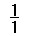÷= ?

Invert the 1/2, creating 2/1, then multiply numerators and denominators to find the answer:x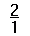==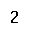Example 2: Fraction division requiring reducing and conversion:÷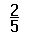= ?

Invert the 2/5, then multiply:x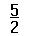=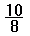Then reduce the fraction:=Then convert the improper fraction to a mixed number:=Got it? Great! Then go to the SuperKids Math Worksheet Creator for Basic Fractions, and give it a try!Questions or comments regarding this site? webmaster@superkids.com